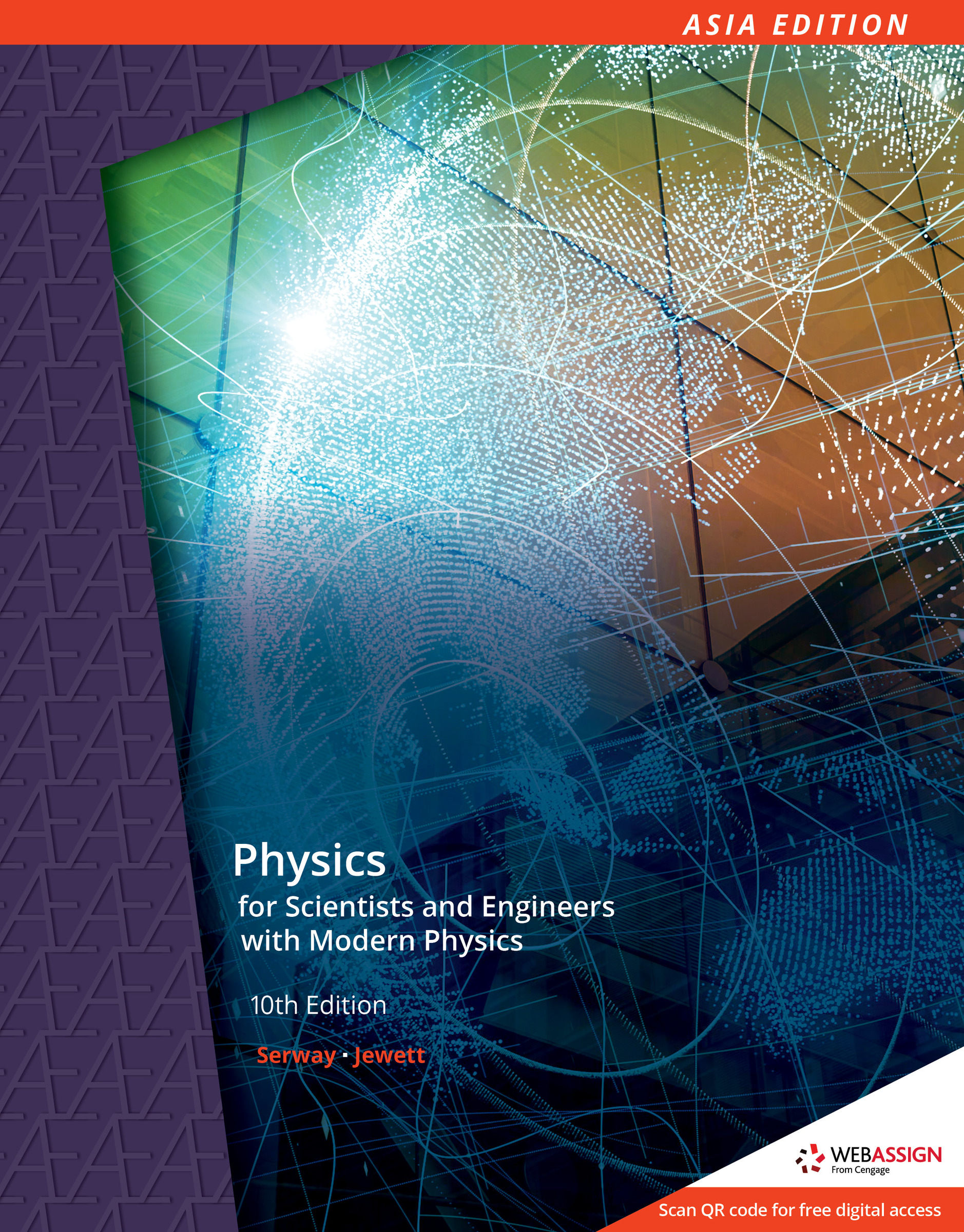# AE PHYSICS FOR SCIENTISTS AND ENGINEERS WITH MODERN PHYSICS RAYMOND SERWAY 9789814834308

RM 120.00

TITLE : AE PHYSICS FOR SCIENTISTS AND ENGINEERS WITH MODERN PHYSICS RAYMOND SERWAY / JOHN JEWETT

ISBN13 : 9789814834308

PUBLISHER : CENGAGE (2018)

EDITION : 10TH ASIA EDITION PAPERBACK

PAGES : 1368 COLOR PAGES

## Overview

Taking an integrative approach, market-leading PHYSICS FOR SCIENTISTS AND ENGINEERS WITH MODERN PHYSICS, Tenth Edition seamlessly matches curated content to the learning environment for which it was intended--from in-class group problem solving to online homework that utilizes targeted feedback and tutorials. More student friendly than ever, the text includes new context-rich exercises, Think-Pair-Share problems, MCAT-style passage problems and sound educational pedagogy. The unified art program and detailed worked examples compliment the concise language and meticulous instruction for which Raymond A. Serway and John W. Jewett Jr. are known. In addition, WebAssign--the world's easiest to use homework system--equips you with the definitive solution to your homework and assessment needs to maximize your course success.

Part I: MECHANICS.
1. Physics and Measurement.
2. Motion in One Dimension.
3. Vectors.
4. Motion in Two Dimensions.
5. The Laws of Motion.
6. Circular Motion and Other Applications of Newton's Laws.
7. Energy of a System.
8. Conservation of Energy.
9. Linear Momentum and Collisions.
10. Rotation of a Rigid Object About a Fixed Axis.
11. Angular Momentum.
12. Static Equilibrium and Elasticity.
13. Universal Gravitation.
14. Fluid Mechanics.
Part II: OSCILLATIONS AND MECHANICAL WAVES.
15. Oscillatory Motion.
16. Wave Motion.
17. Superposition and Standing Waves.
Part III: THERMODYNAMICS.
18. Temperature.
19. Heat and the First Law of Thermodynamics.
20. The Kinetic Theory of Gases.
21. Heat Engines, Entropy, and the Second Law of Thermodynamics.
Part IV: ELECTRICITY AND MAGNETISM.
22. Electric Fields.
23. Continuous Charge Distributions and Gauss's Law.
24. Electric Potential.
25. Capacitance and Dielectrics.
26. Current and Resistance.
27. Direct Current Circuits.
28. Magnetic Fields.
29. Sources of the Magnetic Field.
31. Inductance.
32. Alternating Current Circuits.
33. Electromagnetic Waves.
Part V: LIGHT AND OPTICS.
34. The Nature of Light and the Laws of Geometric Optics.
35. Image Formation.
36. Interference of Light Waves.
37. Diffraction Patterns and Polarization.
Part VI: MODERN PHYSICS.
38. Relativity.
39. Introduction to Quantum Physics.
40. Quantum Mechanics.
41. Atomic Physics.
42. Molecules and Solids.
43. Nuclear Physics.
44. Particle Physics and Cosmology.
APPENDICES.
A. Tables.
B. Mathematics Review.
C. Periodic Table of the Elements.
D. SI Units.
Answers to Quick Quizzes and Odd-Numbered Problems.
Index.